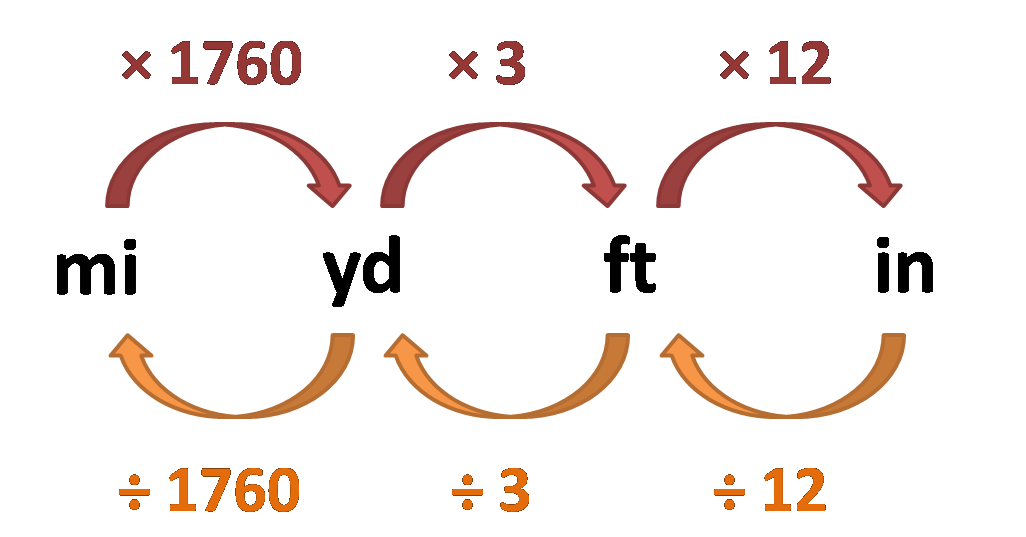# Inch Converter

Created by Maciej Kowalski, PhD candidate
Reviewed by Hanna Pamuła, PhD
Last updated: Feb 02, 2023

Welcome to the inch converter, where we'll see how to convert inches to cm, mm, feet, etc. In essence, it all boils down to multiplying by the right factor, so all we need to do is learn what that number is. Since many of us prefer visuals to text, we present all the data in an elegant table and, in the case of imperial units (e.g., to convert inches to feet), we also give an easy-to-read chart.

## Inch conversion table

As we've already mentioned, going from inches to other units means multiplying by the right factor. In other words, converting inches to mm, converting inches to centimeters, or converting inches to meters is all essentially the same; it's only the number that changes.
In the table below, in each row, we present the decimal number we multiply by when converting to the row's unit.

1 inch (in)

1 millimeter (mm)

304.8

1 centimeter (cm)

30.48

1 meter (m)

0.3048

1 kilometer (km)

0.0003048

1 foot/feet (ft)

12

1 yard (yd)

0.33333

1 mile (mi)

0.0001894

1 nautical mile (nmi)

0.00016458

For instance, converting inches to millimeters requires multiplying by 304.8, so, for example, 3 inches would be
3 in = 3 * 304.8 mm = 914.4 mm.

## Inch conversion chart

In the case of imperial units, the calculations can be exceptionally simple, and all it takes is to understand the chart below.If we start on the far right with inches, we can convert the inches to feet (or, using abbreviations, convert in to ft) by dividing by 12 (the right orange arrow). If we want to go further to yards, we need to additionally divide by 3 (the middle orange arrow). Lastly, converting even further to miles means an extra division by 1760 (the left orange arrow).
For instance, to convert 36 inches to yards would go as follows:
36 in = 36/12 ft = 3 ft = 3/3 yd = 1 yd.

## Other length converters

Remember that the inch converter is not the only tool at your disposal. Below, we list other calculators tailored for all your length conversion needs.

## FAQ

### How do you convert inches to cm?

To convert inches to cm, it's enough to:

1. Take the number of inches you have.
2. Multiply it by 30.48.
3. If needed, round the product to the desired decimal place.
4. Enjoy the result of your conversion.

### How do you convert inches to mm?

In order to convert inches to mm, all you need to do is:

1. Write down the number of inches you got.
2. Multiply it by 304.8.
3. (Optional) Round the product to the desired decimal place.

### How do you convert 108 inches to yards?

To convert 108 inches to yards, we follow these simple steps:

1. Write down the length in inches: 108 in.
2. Divide it by 12 to get feet: 108 in = 108/12 ft = 9 ft.
3. Divide it by 3 to get yards: 9 ft = 9/3 yd = 3 yd.
4. Enjoy knowing that 108 inches is the same as 3 yards.
Maciej Kowalski, PhD candidate
in
Metric
mm
cm
m
km
Imperial/US
ft
yd
mi
Others
nmi
R☉
ly
People also viewed…

### Christmas tree

Welcome to the Christmas tree calculator, where you will find out how to decorate your Christmas tree in the best way. Take a look at the perfect Christmas tree formula prepared by math professors and improved by physicists. Plan in advance how many lights and decorations you'll need!

### Crore to million

The crore to million converter allows you to convert any number in crores to its International place-value counterpart, millions, and vice-versa.

### Helium balloons

Wondering how many helium balloons it would take to lift you up in the air? Try this helium balloons calculator! 🎈

### Lat lon to UTM

This lat long to UTM converter takes geographical latitude and longitude coordinates and shows you the corresponding Universal Transverse Mercator (UTM) coordinate notation.